Question

# B1 a. Let x := 3C1 + 1 and let y := 5C2 + 1. Use...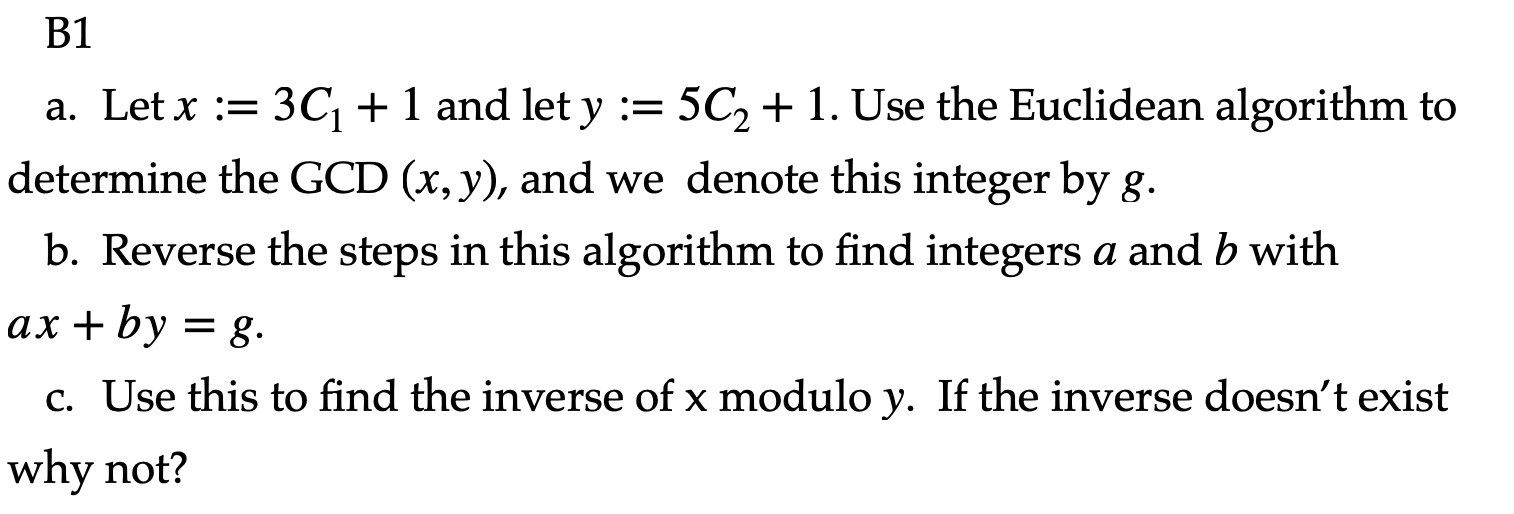B1 a. Let x := 3C1 + 1 and let y := 5C2 + 1. Use the Euclidean algorithm to determine the GCD (x, y), and we denote this integer by g. b. Reverse the steps in this algorithm to find integers a and b with ax+by = 8. c. Use this to find the inverse of x modulo y. If the inverse doesn't exist why not?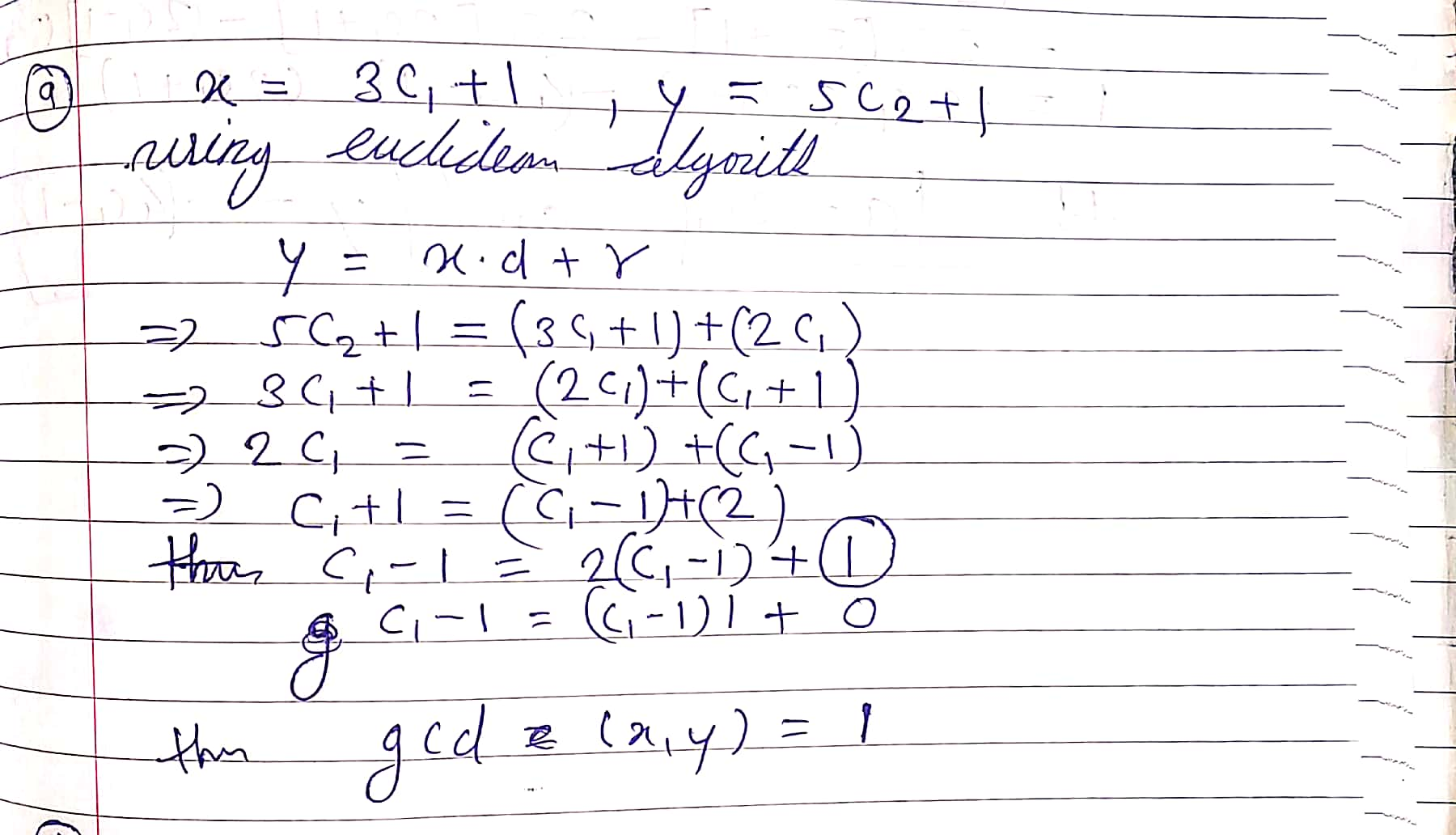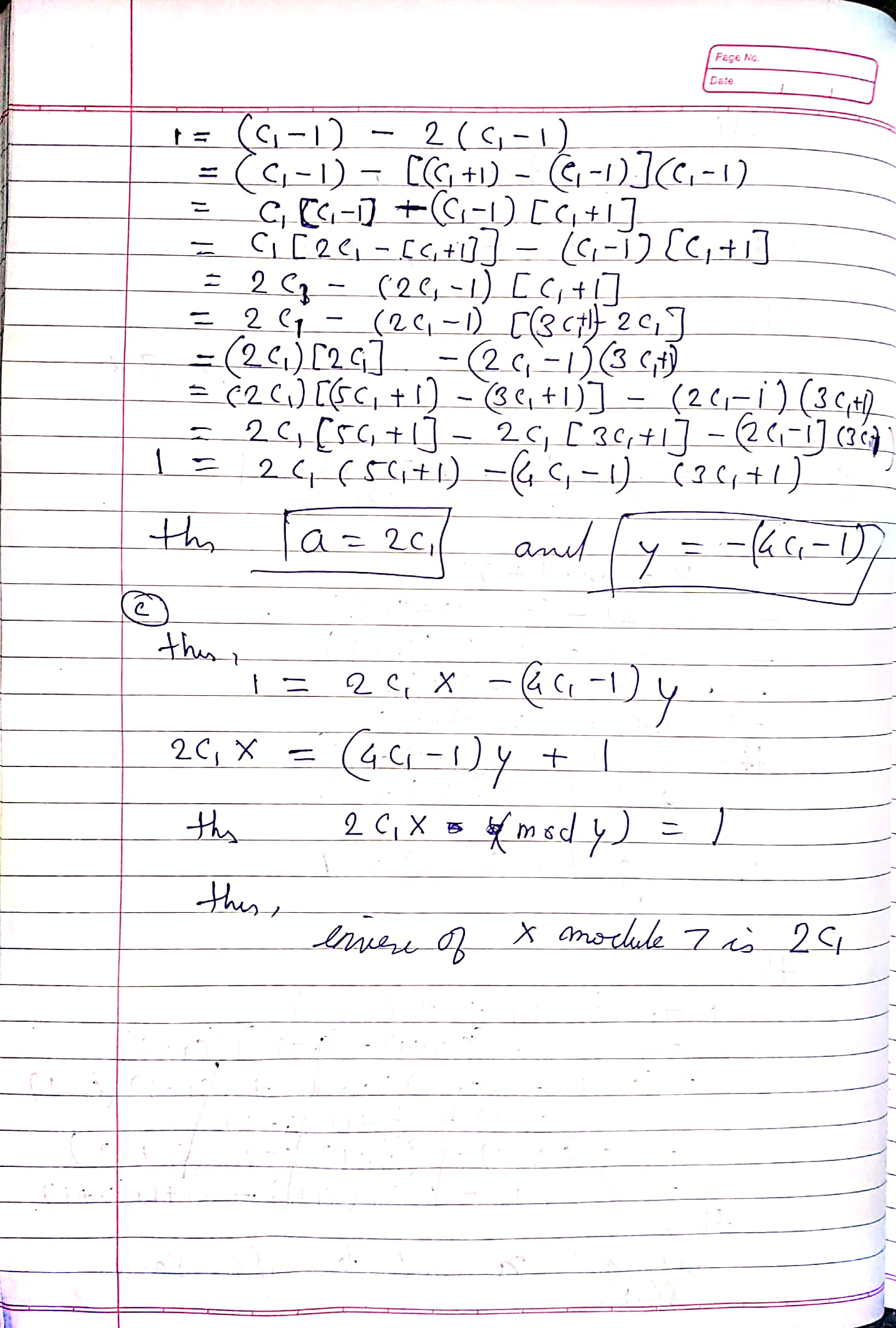#### Earn Coins

Coins can be redeemed for fabulous gifts.

Similar Homework Help Questions
• ### C1= 5 C2= 6 C3= 10 GCD --> Greater Common Divisor B1 a. Let x :=...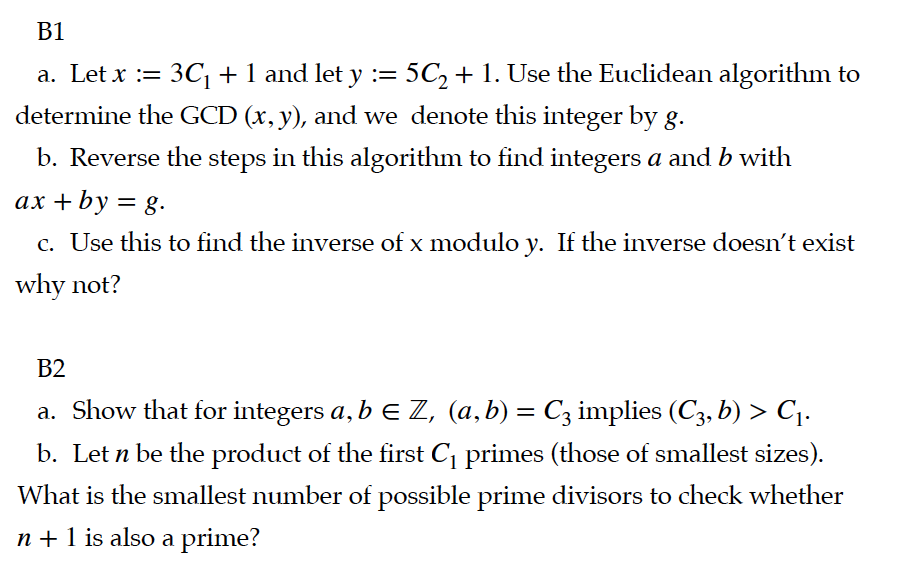C1= 5 C2= 6 C3= 10 GCD --> Greater Common Divisor B1 a. Let x := 3C1 + 1 and let y := 5C2 + 1. Use the Euclidean algorithm to determine the GCD (x, y), and we denote this integer by g. b. Reverse the steps in this algorithm to find integers a and b with ax + by = g. c. Use this to find the inverse of x modulo y. If the inverse doesn't exist why not?...

• ### Required Information Ch 04 Sec 4 EX OG MAIN - Inverse of a modulo m using...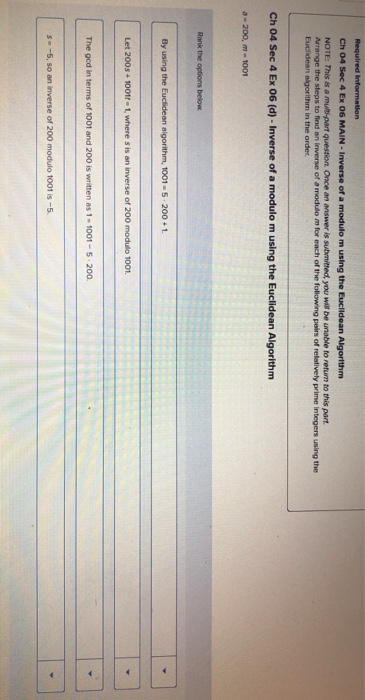Required Information Ch 04 Sec 4 EX OG MAIN - Inverse of a modulo m using the Euclidean Algorithm NOTE: This is a multi-part question. Once an answer is submitted, you will be unable to return to this part. Arrange the steps to find an inverse of a modulo m for each of the following pairs of relatively prime integers using the Euclidean algorithm in the order Ch 04 Sec 4 Ex 06 (d) - Inverse of a modulo m...

• ### Euclidean algorithm 3

Notes: Suppose (a, n) = 1, then there is an integer s such that as = 1 (mod n). This s is called the inverse of a, i.e., a-1 (mod n). The way to find the inverse is asfollows:1) Use the extended Euclidean algorithm to find s and t such that as + nt = 1;2) Then a-1(mod n) = s.In the lecture, we gave an example to find 37-1(mod 121) in three steps.Step-1: use Euclidean algorithm to get the...

• ### Euclidean Algorithm

Notes: Suppose (a, n) = 1, then there is an integer s such that as = 1 (mod n). This s is called the inverse of a, i.e., a-1 (mod n). The way to find the inverse is asfollows:1) Use the extended Euclidean algorithm to find s and t such that as + nt = 1;2) Then a-1(mod n) = s.In the lecture, we gave an example to find 37-1(mod 121) in three steps.Step-1: use Euclidean algorithm to get the...

• ### 1125 and b 56 (a) Find ged(a, b) using: (i) The Euclidean Algorithm (ii) The fundamental...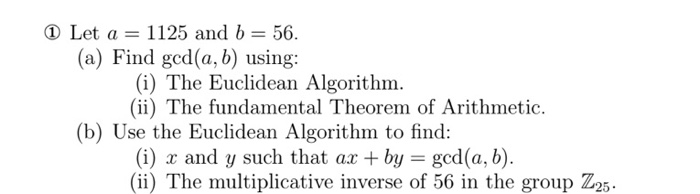1125 and b 56 (a) Find ged(a, b) using: (i) The Euclidean Algorithm (ii) The fundamental Theorem of Arithmetic. (b) Use the Euclidean Algorithm to find: (i) x and y such that ax by ged(a, b) (ii) The multiplicative inverse of 56 in the group Z25. Let a =

• ### Using the Euclidean Algorithm show that gcd (193, 977) Now find integers s, t such that 193s +977t-1, and use this to find the value of a that satisfies the congruence 193a 38 (mod 977) Using th...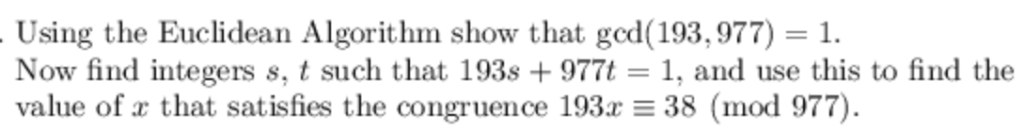Using the Euclidean Algorithm show that gcd (193, 977) Now find integers s, t such that 193s +977t-1, and use this to find the value of a that satisfies the congruence 193a 38 (mod 977) Using the Euclidean Algorithm show that gcd (193, 977) Now find integers s, t such that 193s +977t-1, and use this to find the value of a that satisfies the congruence 193a 38 (mod 977)

• ### 6. Using the Euclidean Algorithm show that gcd (109, 736) 1 Now find integers s, t such that 109s + 736t 1, and use this to find the value of r that satisfies the congruence 109x 71 (mod 736). 6...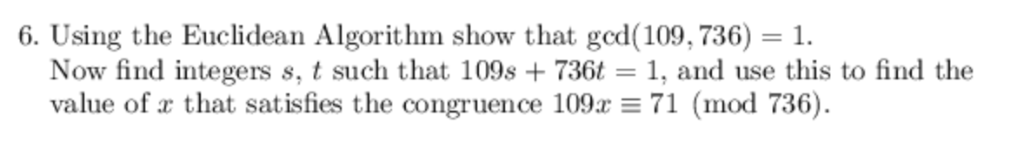6. Using the Euclidean Algorithm show that gcd (109, 736) 1 Now find integers s, t such that 109s + 736t 1, and use this to find the value of r that satisfies the congruence 109x 71 (mod 736). 6. Using the Euclidean Algorithm show that gcd (109, 736) 1 Now find integers s, t such that 109s + 736t 1, and use this to find the value of r that satisfies the congruence 109x 71 (mod 736).

• ### Let a and be be in . Show the following. If gcd(a,b)=1, then for every n...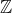Let a and be be in . Show the following. If gcd(a,b)=1, then for every n in there exist x and y in such that n=ax+by. We were unable to transcribe this imageWe were unable to transcribe this imageWe were unable to transcribe this image

• ### PROBLEM 1 For each of the following pairs of integers, use the Euclidean Algorithm to find...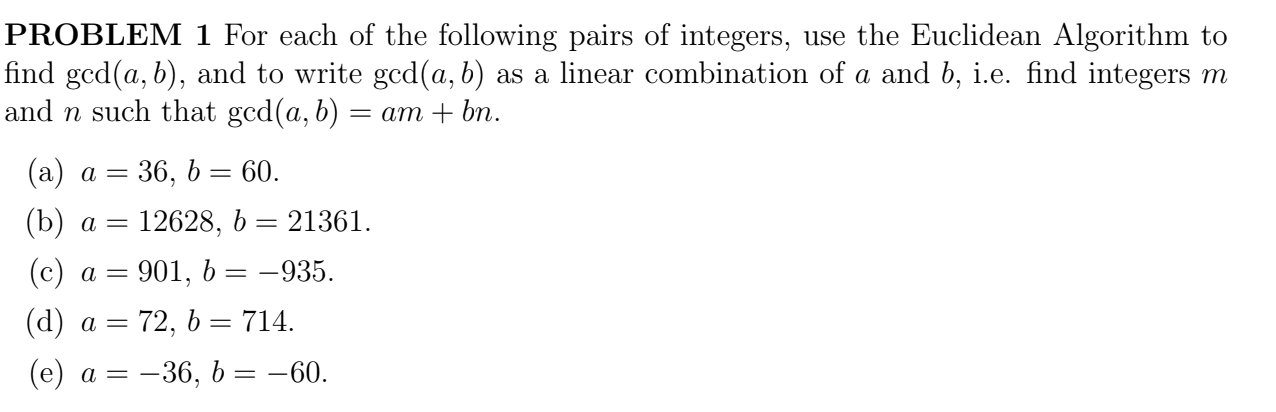PROBLEM 1 For each of the following pairs of integers, use the Euclidean Algorithm to find ged(a,b), and to write gcd(a,b) as a linear combination of a and b, i.e. find integers m and n such that gcd(a,b) = am + bn. (a) a = 36, b = 60. (b) a = 12628, b = 21361. (c) a = 901, b = -935. (d) a = 72, b = 714. (e) a = -36, b = -60.

• ### 2. Let n be a positive integer. Denote the number of positive integers less than n and rela- tive...number thoery just need 2 answered 2. Let n be a positive integer. Denote the number of positive integers less than n and rela- tively prime to n by p(n). Let a, b be positive integers such that ged(a,n) god(b,n)-1 Consider the set s, = {(a), (ba), (ba), ) (see Prollern 1). Let s-A]. Show that slp(n). 1. Let a, b, c, and n be positive integers such that gcd(a, n) = gcd(b, n) = gcd(c, n) = 1 If...